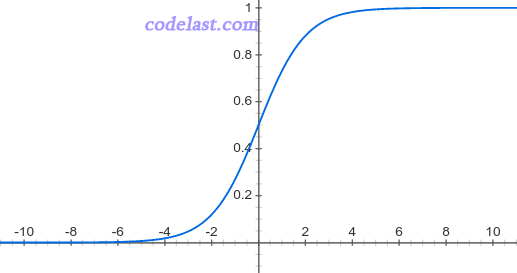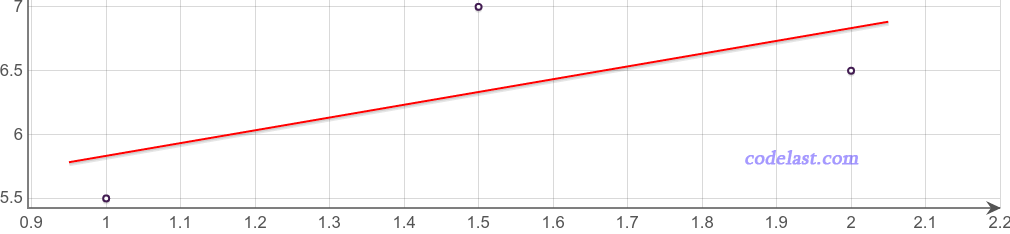# [原创] 用人话解释机器学习中的Logistic Regression（逻辑回归）

Logistic Regression（或Logit Regression），即逻辑回归，简记为LR，是机器学习领域的一种极为常用的算法／方法／模型。

Logistic Regression是一种分类算法。分类，也就是把一个群体（或问题，或数据）分为几个类别，例如，男/女/人妖；爱她的人/不爱她的人；今天会下雨/今天不会下雨。
Logistic Regression最常用于处理“二分类”问题，也就是说分类只有两个，像“爱她的人/不爱她的人”就是二分类，而“男/女/人妖”就不是二分类。当然，Logistic Regression也可以用于处理多分类问题，即所谓的“多分类逻辑回归”（Multiclass Logistic Regression），但本文并不涉及这个方面。

Logistic Regression在现实世界中非常有用。例如，可以用它来判断一个用户是否会点击一个广告（会点击／不会点击），可以用Logistic Regression来判断两类人是否会相爱（会相爱／不会相爱），等等。

(1, 5.5)
(1.5, 7)
(2, 6.5)

Logistic Regression也是类似，我们有一个函数 $y = f(X)$ ，里面包含若干个未知参数 ${\theta _0},{\theta _1},{\theta _2}, \cdots ,{\theta _n}$

$f(X) = \frac{1}{{1 + {e^{ - {\theta ^T}X}}}}{\rm{ }}$ ……（1）

$s = \sum\limits_{i = 0}^n {{\theta _i}{x_i}} = {\theta _0}{x_0} + {\theta _1}{x_1} + \cdots + {\theta _n}{x_n} = {\theta ^T}X$

$P(t) = \frac{1}{{1 + {e^{ - t}}}}$$y' = \left\{ {\begin{array}{*{20}{c}}{0,f(X) > 0.5}\\{1,f(X) \le 0.5}\end{array}} \right.$

(1, 5.5)
(1.5, 7)
(2, 6.5)• 损失函数Loss Function代价函数Cost Function

WiKi的定义：
In mathematical optimization, statistics, decision theory and machine learning, a loss function or cost function is a function that maps an event or values of one or more variables onto a real number intuitively representing some "cost" associated with the event. An optimization problem seeks to minimize a loss function.

The loss function quantifies the amount by which the prediction deviates from the actual values.

（1）0-1损失函数：可用于分类问题，即该函数用于衡量分类错误的数量，但由于此损失函数是非凸（non-convex）的，因此在做最优化计算时，难以求解，所以，正因为如此，0-1损失函数不是那么“实用”（如果这句话有误，请指正）。
（2）平方损失函数（Square Loss）：常用于线性回归（Linear Regression）。
（3）对数损失（Log Loss）函数：常用于其模型输出每一类概率的分类器（classifier），例如逻辑回归。
（4）Hinge损失函数：常用于SVM（Support Vector Machine，支持向量机，一种机器学习算法）。中文名叫“合页损失函数”，因为hinge有“合页”之意。这个翻译虽然直白，但是你会发现，99％的文章都不会用它的中文名来称呼它，而是用“Hinge损失”之类的说法。

$L = - \frac{1}{n}\sum\limits_{i = 1}^n {[{y_i}\log ({{\hat y}_i}) + (1 - {y_i})\log (1 - {{\hat y}_i})]}$ ......（2）

$L = - \frac{1}{N}\sum\limits_{i = 1}^n {\left[ {{y_i}\log \left( {\frac{1}{{1 + {e^{ - {\theta ^T}{X_i}}}}}} \right) + (1 - {y_i})\log \left( {1 - \frac{1}{{1 + {e^{ - {\theta ^T}{X_i}}}}}} \right)} \right]}$

$L(\theta ) = - \frac{1}{N}\sum\limits_{i = 1}^n {\left[ {{y_i}\log \left( {\frac{1}{{1 + {e^{ - {\theta ^T}{X_i}}}}}} \right) + (1 - {y_i})\log \left( {1 - \frac{1}{{1 + {e^{ - {\theta ^T}{X_i}}}}}} \right)} \right]}$

• 求解方法

➤➤ 版权声明 ➤➤### 《[原创] 用人话解释机器学习中的Logistic Regression（逻辑回归）》有18条评论

1. 上data anlysis时讲到logistic regression，实在是听不懂印度教授讲的内容，看此文胜读十年书，感谢。

回复
2. Thank you for your extremely detailed explanation Darran! It helps me a lot.

回复
3. 非常棒的讲解，让人豁然开朗

回复
4. 非常感谢博主，没有数学基础的小白终于搞懂了！

回复
5. 非常好 很感谢

回复
6. 博主讲的很清晰易懂啊 之前对对数损失函数和交叉熵有疑惑 现在懂了

回复
7. Excellent 1!! 希望能看到更多机械学习的文章

回复
8. 收获太多，感谢作者分享

回复
9. 人話 讓人類更好理解
感謝作者!

回复
10. 万谢，解答了我的疑惑，博主加油哦

回复
11. 看了你的评论我对我来说就是醍醐灌顶 谢谢！ 很想认识你

回复
12. 您寫得太好了, 讓我心領神會。有個問題想再請教一下，關於θ訓練出來之後帶入式子1, 是要用什麼門檻值來決定是屬於第1類還是屬於2類呢? 還是通常都使用0.5 呢?

回复
• 这个阈值是根据业务的实际情况来设置／调整的，并不是个固定值，需要根据数据来分析。

回复
13. 你的答案就是我想要的

回复
14. 发现了一个干货博客，好玩的内容也很多，好开心，收藏之！

回复
15. 您写的非常好。

回复